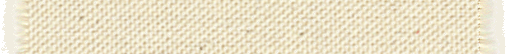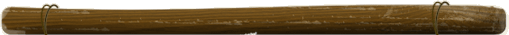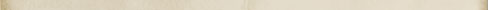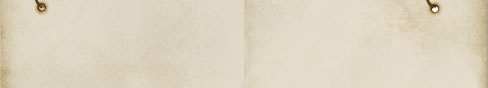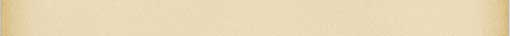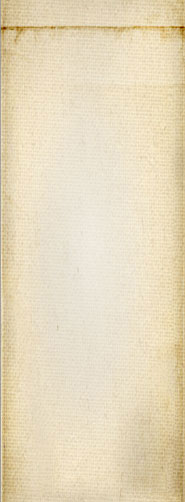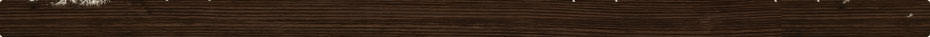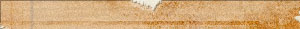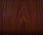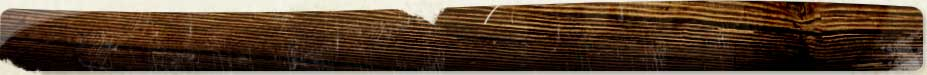# The if() and conditional operator (?:) in CSS Preprocessor

The CSS Preprocessor adds the capability to write complex expressions and functions to use in your CSS files.

One of the functions you can use is a system function named if. It is used to select one of two expressions as shown here:

`if(\$color = white, 33px, 145px)`

This says if \$color represents the color white, then use 33px, otherwise use 145px.

This works great in all cases where the true and false expressions are both calculable. If one of the expressions is to generate an error, then the if() function cannot be used. In this case, you want to use the ?: operator instead.

In the following, the second member generates a division by zero error. However, because the test is true (red(\$color) = 0), the operator ignores the error in the third expression and moves on.

`red(\$color) = 0 ? 0 : 7 / red(\$color)`

With really complicated expressions, this may not work as expected. This is because the expression parser stops short and thus you may get other errors. We will look into a better way at some point to actually transform expressions in a tree before executing them. That way we can even completely ignore the right hand side in this very case! This is part of our TODO list...Snap! Websites
An Open Source CMS System in C++